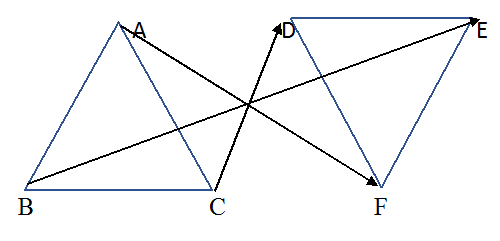# Ex.7.1 Q3 Congruence of Triangles - NCERT Maths Class 7

Go back to  'Ex.7.1'

## Question

If $$ΔABC ≅ ΔFED$$ under the correspondence $$ABC ↔ FED,$$ write all the corresponding congruent parts of the triangles.

Video Solution
Congruence Of Triangles
Ex 7.1 | Question 3

## Text Solution

What is the known?

Two triangles which are congruent.

What is the unknown?

Triangles $$ABC$$ and $$FED$$ are congruent $$(ΔABC ≅ ΔFED)$$ under the correspondence $$ABC ↔ FED$$

Reasoning:

Two congruent triangles have exactly the same three sides and exactly the same three angles. According to the given correspondence, triangle$$ABC$$ can be placed on triangle$$FED$$ in such a way that $$A$$ falls on $$F$$, $$B$$ falls on $$E$$ and $$C$$ falls on $$D$$. So, while taking about congruence of triangles, measure of sides, measure of angles and matching of vertices are equally important.

Steps:

Given, $$ΔABC ≅ ΔFED$$ under the correspondence $$ABC ↔ FED.$$ The correspondence is shown with arrow in the following figure.It is clear from the figure that

\begin{align}& \angle A\leftrightarrow \! \angle F,\!\angle \! B\leftrightarrow \! \angle E, \!\angle C \! \leftrightarrow \! \angle D \\& \overline{AB}\ \! \leftrightarrow \! \overline{FE}, \!\overline{BC}\ \! \leftrightarrow \! \overline{ED}, \!\overline{AC}\ \! \leftrightarrow \! \overline{FD} \\ \end{align}

Learn from the best math teachers and top your exams

• Live one on one classroom and doubt clearing
• Practice worksheets in and after class for conceptual clarity
• Personalized curriculum to keep up with school